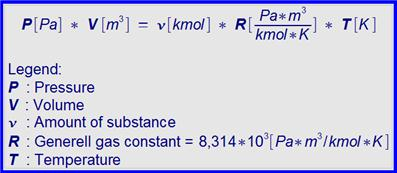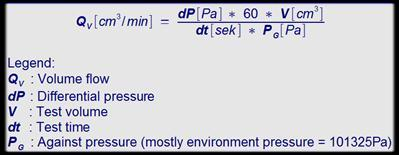# Pressure change measure - flow rate spend

With some formulas (Black frame) the appropriate on-line computation assistance can be opened by clicking on the formula window. Basis of the leak test with compressed air is the “general gas law”:By an appropriate derivation one finally arrives at the formula at the determination of the flow rate from the measured decrease of pressure and/or increase of pressure. Here it is accepted that during the examination the mass and the temperature remain constant. In this case the result calls volume flow.

In the resulting formula the test volume of the complete test equipment and the pressure are against that the leakage work contained.  Only so the leakage can be determined as volume flow precisely:In this formula the test pressure and the gas temperature is not included. This connection must be manufactured separately. All devices with the differential pressure method work according to this principle.

Sample 1: Computation of a leakage from lost pressure

Lost pressure dP = 100 Pa; Test volume V = 2000 cm3; Measurement time dt = 5 sek

QV = (100 * 60 * 2000) / (5 * 101325) = 23,69 cm3/min

Sample 2: Computation of the minimum measurement time with given dispersion

Lost pressure dP (=minimum dispersion) = 5 Pa; Test volume V = 5000 cm3; Volume flow (=leakage) QV = 10 cm3/min

dt = (5 * 60 * 5000) / (10 * 101325) = 1,48 sek